# 635 Some Multiplication Facts to Know Backwards and Forwards

Something amazing happens in the multiplication table when an even number is multiplied by 6.

Something just as amazing (but backwards) happens in the multiplication table when a multiple of 3 is multiplied by 7.

Here is a graphic that may be helpful for students memorizing some of those 6 and 7 multiplication facts. It is meant to be read from left to right and contains some cool coincidences: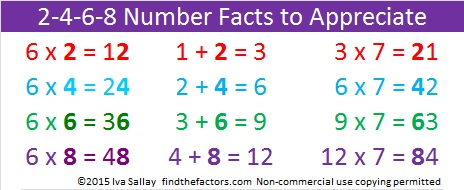Knowing those 6 and 7 multiplication facts will help any student know the multiplication table backwards and forwards!

While it isn’t necessary to memorize the following number facts, some of the patterns in the graphic above continue with them:

• 10 + 5 = 15, and 15 x 7 = 105
• 12 + 6 = 18, and 18 x 7 = 126
• 14 + 7 = 21, and 21 x 7 = 147
• 16 + 8 = 24, and 24 x 7 = 168
• 18 + 9 = 27, and 27 x 7 = 189

———————————————————

What makes 635 an interesting number?

635 is the sum of the nine prime numbers from 53 to 89.

It is also the sum of the thirteen prime numbers from 23 to 73.

635 can be written as the sum of 5 consecutive numbers:

125 + 126 + 127 + 128 + 129 = 635.

635 is a part of exactly 4 Pythagorean triples. Which factors of 635 are greatest common factors for the non-primitive triples?

• 635 is the hypotenuse of the Pythagorean triple 381-508-635
• 635 is the short leg in the triple 635-1524-1650
• 635 is the short leg in the triple 635-40320-40325
• 635 is the short leg in the primitive triple 635-201612-201613

———————————————————————–

• 635 is a composite number.
• Prime factorization: 635 = 5 x 127
• The exponents in the prime factorization are 1 and 1. Adding one to each and multiplying we get (1 + 1)(1 + 1) = 2 x 2 = 4. Therefore 635 has exactly 4 factors.
• Factors of 635: 1, 5, 127, 635
• Factor pairs: 635 = 1 x 635 or 5 x 127
• 635 has no square factors that allow its square root to be simplified. √635 ≈ 25.199206.# 199 My List of Multiplication Rhymes

• 199 is a prime number.
• Prime factorization: 199 is prime.
• The exponent of prime number 199 is 1. Adding 1 to that exponent we get (1 + 1) = 2. Therefore 199 has exactly 2 factors.
• Factors of 199: 1, 199
• Factor pairs: 199 = 1 x 199
• 199 has no square factors that allow its square root to be simplified. √199 ≈ 14.1067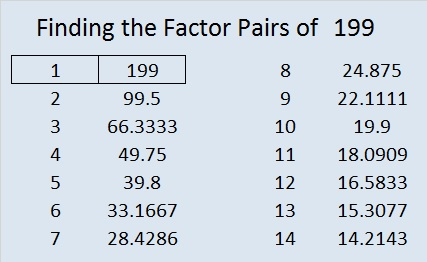How do we know that 199 is a prime number? If 199 were not a prime number, then it would be divisible by at least one prime number less than or equal to √199 ≈ 14.1. Since 199 cannot be divided evenly by 2, 3, 5, 7, 11, or 13, we know that 199 is a prime number.

———————————

I recently wrote a post that asked “Are Multiplication Rhymes Able to Help Kids Learn the Multiplication Table?” Here is a list of rhymes that I’ve put together.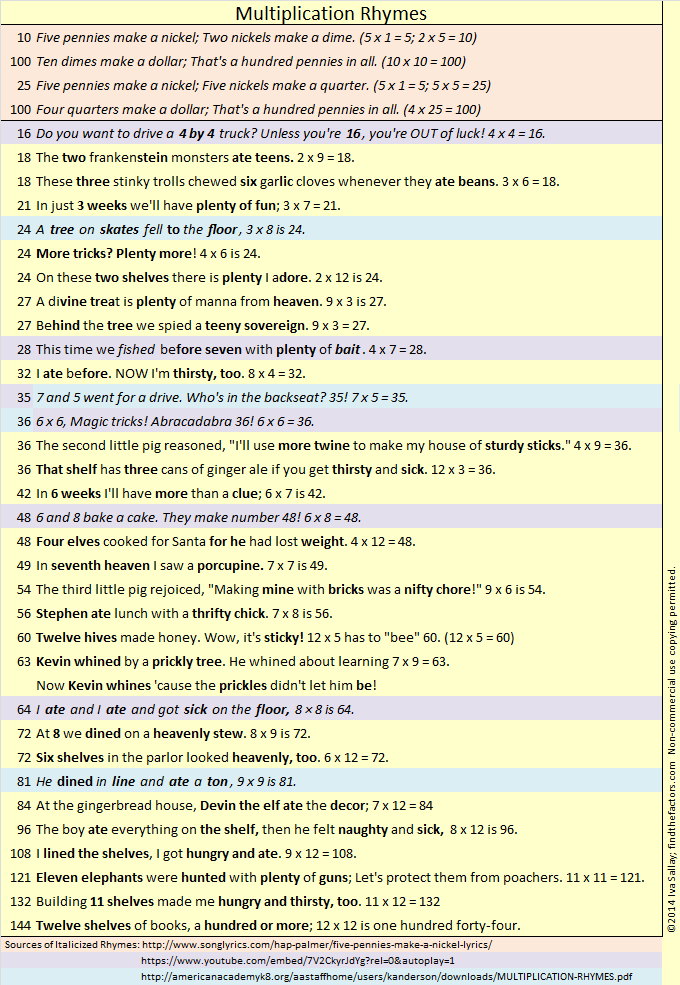Most of the rhymes have rhyming clues to help students remember both numbers being multiplied as well as their product. I wrote the rhymes that are highlighted in yellow. Those highlighted in peach, purple, or blue are from other sources, but I did alter the rhymes for 24, 28, and 81. A black and white pdf of the rhymes is also available here.

# 194 Are Multiplication Rhymes Able to Help Kids Learn the Multiplication Table?

194 is a composite number. Factor pairs: 194 = 1 x 194 or 2 x 97. Factors of 194: 1, 2, 97, 194. Prime factorization: 194 = 2 x 97.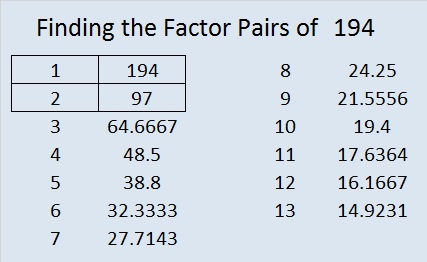Are multiplication rhymes able to help kids learn the multiplication table? The obvious answer is, of course, YES! Duh!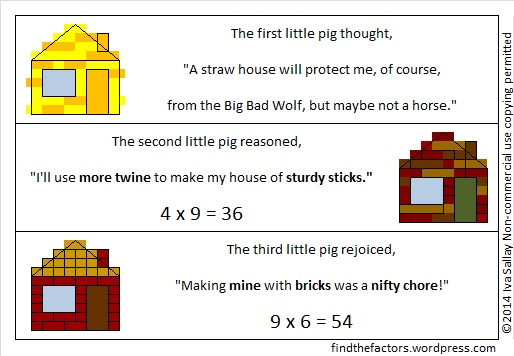However, sometimes this obvious answer may not be correct. I recently read a researched article on the effectiveness of certain teaching practices in helping first-grade children learn mathematics. The points made in the article would likely apply to third and fourth-grade students learning multiplication as well. The article states that alternative techniques (music, movement, m&m’s, other manipulatives, etc.) do reinforce concepts for students who already understand what is being taught, but students who struggle actually make no gains when unproven techniques are used. The researcher hypothesized that when students struggle to learn mathematical concepts “alternative techniques tend to demand more, cognitively, from [these] students.” Demanding more cognitively can backfire: Requiring a student to memorize a rhyme and the math fact could put that student’s brain on overload with even fewer facts learned.

I have now modified my opinion of multiplication rhymes. If rhyme-and-rhythm is helpful, use it, if not, don’t. What is good for some of the class, may not be good for all of the class. If a student doesn’t make progress using the rhymes, it may be better to stick with more direct instruction to learn the math facts. Additionally, each rhyme should be examined individually and its own pros and cons considered.

Here are two of my FAVORITE sources of multiplication rhymes: American Academy’s MULTIPLICATION-RHYMES.pdf now requires registration to view, but it has rhymes printed in pretty colors and contains a few of my favorite multiplication rhymes. Kids would probably like looking at this pdf multiple times.

Multiplication Rhymes 1 is an entertaining You-tube video made by Mrs. Rice that children would probably enjoy watching over and over again, and it also has a few very memorable rhymes.

Although these two sources are great, I think some of the rhymes are too similar:

• From the pdf, we have “8 and 4 were sad and blue, 8 x 4 = 32” while the video recites “6 and 7 are sad and blue, they make number 42.” If students learned either of those rhymes, they may have difficulty remembering the product or which two numbers were sad and blue several weeks later.
• The video also gave two other rhymes that were too similar to each other: “9 and 8 what do they do? They go play (tag) with 72.” and  “9 and 9 are having fun. They play tag with 81.” “Tag” is in parentheses because it wasn’t on the note card that was shown, but it was spoken on the video. Again weeks later, groups or individuals may not remember which numbers were playing tag or what the product was when they were multiplied together.

I compiled my own List of Multiplication Rhymes which focus on the multiplication facts highlighted in the multiplication table below. I included some rhymes from the above pdf and the video and added some from the song Five Pennies Make a Nickel. All of those rhymes are in italics. The rhymes that I wrote myself are in regular print. If I modified an existing rhyme, the modifications are in regular print while the rest of the rhyme is in italics. I also ordered the rhymes in a way that should make finding the rhyme for any particular fact much easier. I hope there will be many rhymes on this list that you enjoy as well, and that they get used to help many students learn the very important multiplication facts highlighted in the table below.